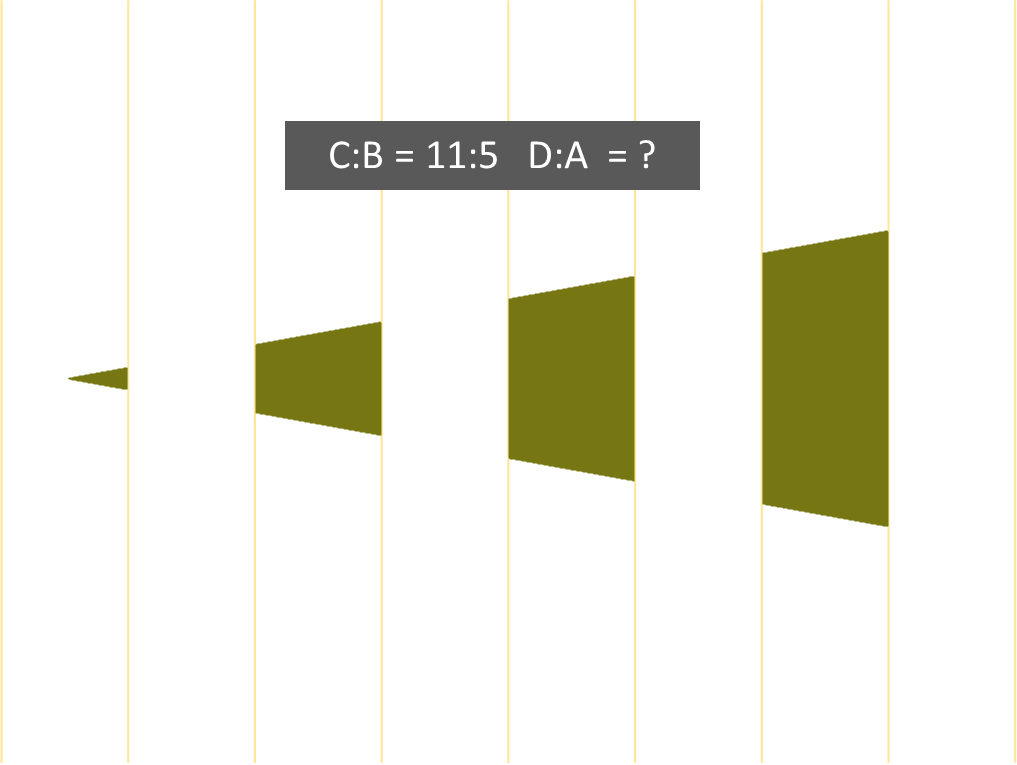# AIME Math Puzzle

Here's a fun little puzzle that came from an AIME exam paper in 2006:
Problem: In the diagram below, a triangle is drawn over a series of, equally-spaced, parallel lines. If the ratio of the regions C:B is 11:5, what is the ratio of the regions D:A?
Note: The apex of the triangle is not on any of the lines.What is the ratio D:A?